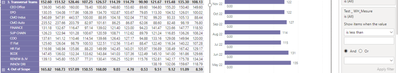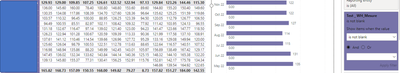cancel
Showing results for
Did you mean:Helper II

## Filter Blank values in a division without using filter Panel

Hi All,

I have created a DAX expression to calculate an average based on a division, my issue is to have the correct figures I need to remove from the calculation blank value, the only Solution that I found so far it is to use the filter pane and I would like to know if I can filter the value inside my DAX expression :

``````Test _ WH_Mesure =

VAR Numerator =
CALCULATE(
AVERAGE('Fact Table'[Value]),
FILTER(ALL(DimWHAttribute[Attribute]), DimWHAttribute[Attribute] = "Attribute A"
)
)

VAR Denominator =
CALCULATE(
AVERAGE('Fact Table'[Value]),
FILTER(ALL(DimWHAttribute[Attribute]), DimWHAttribute[Attribute] = "Attribute B"
)
)

VAR DivisionResult =
DIVIDE(Numerator, Denominator, 0)

RETURN
SWITCH(
TRUE(),
ISBLANK(DivisionResult) || DivisionResult = 0 || ISBLANK(Numerator) || ISBLANK(Denominator),
BLANK(),
DivisionResult
)``````Memorable Member

Hi @Fantmas if not already, check article https://www.sqlbi.com/articles/blank-handling-in-dax/ .

Keep in mind how DAX engine treat blank in functions like AVERAGE, link https://learn.microsoft.com/en-us/dax/average-function-dax .

It could be that you need to use AVERAGEX function. I hope this help.

Sarajevo, Bosnia and Herzegovina